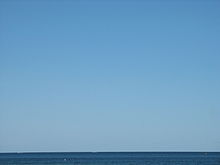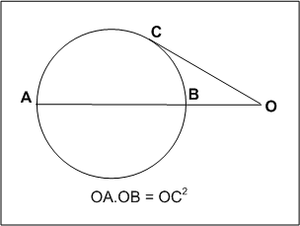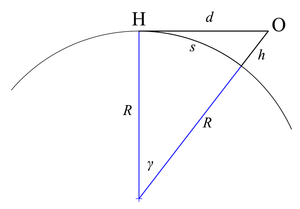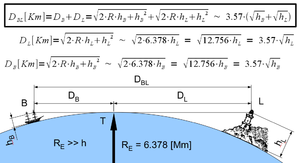# Horizon

﻿
HorizonA water horizon, in northern Wisconsin, U.S.

The horizon (or skyline) is the apparent line that separates earth from sky, the line that divides all visible directions into two categories: those that intersect the Earth's surface, and those that do not. At many locations, the true horizon is obscured by trees, buildings, mountains, etc., and the resulting intersection of earth and sky is called the visible horizon. When looking at a sea from a shore, the part of the sea closest to the horizon is called the offing. The word horizon derives from the Greek "ὁρίζων κύκλος" (horizōn kyklos), "separating circle", from the verb "ὁρίζω" (horizō), "to divide, to separate", and that from "ὅρος" (oros), "boundary, landmark".

## Appearance and usageView of Earth's horizon as seen from Space Shuttle Endeavour, 2002

Historically, the distance to the visible horizon at sea has been extremely important as it represented the maximum range of communication and vision before the development of the radio and the telegraph. Even today, when flying an aircraft under Visual Flight Rules, a technique called attitude flying is used to control the aircraft, where the pilot uses the visual relationship between the aircraft's nose and the horizon to control the aircraft. A pilot can also retain his or her spatial orientation by referring to the horizon.

In many contexts, especially perspective drawing, the curvature of the earth is disregarded and the horizon is considered the theoretical line to which points on any horizontal plane converge (when projected onto the picture plane) as their distance from the observer increases. For observers near sea level the difference between this geometrical horizon (which assumes a perfectly flat, infinite ground plane) and the true horizon (which assumes a spherical Earth surface) is imperceptible to the naked eye (but for someone on a 1000-meter hill looking out to sea the true horizon will be about a degree below a horizontal line).

In astronomy the horizon is the horizontal plane through (the eyes of) the observer. It is the fundamental plane of the horizontal coordinate system, the locus of points that have an altitude of zero degrees. While similar in ways to the geometrical horizon, in this context a horizon may be considered to be a plane in space, rather than a line on a picture plane.

## Distance to the horizon

The approximate distance to the horizon from an observer close to the Earth's surface is given by$d \approx 3.856\sqrt{h} \,,$

where d is in kilometres and h is height above sea level in metres.

Examples:

• For an observer standing on the ground with h = 1.70 metres (5 ft 7 in) (average eye-level height), the horizon is at a distance of 5.0 kilometres (3.1 mi).
• For an observer standing on a hill or tower of 100 metres (330 ft) in height, the horizon is at a distance of 39 kilometres (24 mi).
• For an observer standing at the top of the Burj Khalifa (828 metres (2,717 ft) in height), the horizon is at a distance of 111 kilometres (69 mi).

With d in miles and h in feet,$d \approx 1.323\sqrt{h} \,.$

Examples:

• For an observer on the ground with eye level at h = 5 ft 7 in (5.583 ft), the horizon is at a distance of 3.1 miles (5.0 km).
• For an observer standing on a hill or tower 100 feet (30 m) in height, the horizon is at a distance of 13.2 miles (21.2 km).
• For an observer on the summit of Aconcagua (22,841 feet (6,962 m) in height), the sea-level horizon to the west is at a distance of 200 miles (320 km).

These formulas include the estimated effect of atmospheric refraction.

### Geometrical modelGeometrical basis for calculating the distance to the horizon, secant tangent theoremGeometrical distance to the horizon, Pythagorean theoremThree types of horizon

If the Earth is assumed to be a perfect sphere with no atmosphere, then the theoretical formula for the distance of the horizon can easily be calculated using Euclidean geometry.

The secant tangent theorem states that$\mathrm{OC}^2 = \mathrm{OA} \times \mathrm{OB} \,.$

Make the following substitutions:

• d = OC = distance to the horizon
• D = AB = diameter of the Earth
• h = OB = height of the observer above sea level
• D+h = OA = diameter of the Earth plus height of the observer above sea level

The formula now becomes$d^2 = h(D+h)\,\!$

or$d = \sqrt{h(D+h)} =\sqrt{h(2R+h)}\,,$

where R is the radius of the Earth.

Alternatively, this equation can be derived using the Pythagorean theorem. Since the line of sight is a tangent to the Earth, it is perpendicular to the radius at the horizon. This sets up a right triangle, with the sum of the radius and the height as the hypotenuse. With

• d = distance to the horizon
• h = height of the observer above sea level
• R = radius of the Earth

referring to the second figure at the right leads to the following:$(R+h)^2 = R^2 + d^2 \,\!$$R^2 + 2Rh + h^2 = R^2 + d^2 \,\!$$d = \sqrt{h(2R + h)} \,.$

Another relationship involves the distance s along the curved surface of the Earth to the horizon; with γ in radians,$s = R \gamma \,;$

then$\cos \gamma = \cos\frac{s}{R}=\frac{R}{R+h}\,.$

Solving for s gives$s=R\cos^{-1}\frac{R}{R+h} \,.$

The distance s can also be expressed in terms of the line-of-sight distance d; from the second figure at the right,$\tan \gamma = \frac {d} {R} \,;$

substituting for γ and rearranging gives$s=R\tan^{-1}\frac{d}{R} \,.$

The distances d and s are nearly the same when the height of the object is negligible compared to the radius (that is, h ≪ R).

### Approximate geometrical formulas

If the observer is close to the surface of the earth, then it is valid to disregard h in the term (2R + h), and the formula becomes$d = \sqrt{2Rh} \,.$

Using metric units and taking the radius of the Earth as 6371 km, the distance to the horizon is$d \approx \sqrt{12.74h} \approx 3.57\sqrt{h} \,,$

where d is in kilometres, and h is the height of the eye of the observer above ground or sea level in metres.

Using imperial units, the distance to the horizon is$d \approx \sqrt{1.50h} \approx 1.22 \sqrt{h} \,,$

where d is in miles and h is in feet.

These formulas may be used when h is much smaller than the radius of the Earth (6371 km), including all views from any mountaintops, aeroplanes, or high-altitude balloons. With the constants as given, both the metric and imperial formulas are precise to within 1% (see the next section for how to obtain greater precision).

### Exact formula for a spherical Earth

If h is significant with respect to R, as with most satellites, then the approximation made previously is no longer valid, and the exact formula is required:$d = \sqrt{2Rh + h^2} \,,$

where R is the radius of the Earth (R and h must be in the same units). For example, if a satellite is at a height of 2000 km, the distance to the horizon is 5,430 kilometres (3,370 mi); neglecting the second term in parentheses would give a distance of 5,048 kilometres (3,137 mi), a 7% error.

### Objects above the horizonGeometrical horizon distance

To compute the height of an object visible above the horizon, compute the distance to the horizon for a hypothetical observer on top of that object, and add it to the real observer's distance to the horizon. For example, for an observer with a height of 1.70 m standing on the ground, the horizon is 4.65 km away. For a tower with a height of 100 m, the horizon distance is 35.7 km. Thus an observer on a beach can see the tower as long as it is not more than 40.35 km away. Conversely, if an observer on a boat (h = 1.7 m) can just see the tops of trees on a nearby shore (h = 10 m), the trees are probably about 16 km away.

Referring to the figure at the right, the lighthouse will be visible from the boat if$D_\mathrm{BL} < 3.57\,(\sqrt{h_\mathrm{B}} + \sqrt{h_\mathrm{L}}) \,,$

where DBL is in kilometres and hB and hL are in metres. If atmospheric refraction is considered, the visibility condition becomes$D_\mathrm{BL} < 3.86\,(\sqrt{h_\mathrm{B}} + \sqrt{h_\mathrm{L}}) \,.$

### Effect of atmospheric refraction

Because of atmospheric refraction of light rays, the actual distance to the horizon is slightly greater than the distance calculated with geometrical formulas. With standard atmospheric conditions, the difference is about 8%; however, refraction is strongly affected by temperature gradients, which can vary considerably from day to day, especially over water, so calculated values for refraction are only approximate.

Rigorous method—Sweer
The distance d to the horizon is given by$d={{R}_{\text{E}}}\left( \psi +\delta \right) \,,$

where RE is the radius of the Earth, ψ is the dip of the horizon and δ is the refraction of the horizon. The dip is determined fairly simply from$\cos \psi =\frac{{{R}_{\text{E}}}{{\mu }_{0}}}{\left( {{R}_{\text{E}}}+h \right)\mu } \,,$

where h is the observer's height above the Earth, μ is the index of refraction of air at the observer's height, and μ0 is the index of refraction of air at Earth's surface.

The refraction must be found by integration of$\delta =-\int_{0}^{h}{\tan \phi \frac{\text{d}\mu }{\mu }} \,,$

where$\phi\,\!$ is the angle between the ray and a line through the center of the Earth. The angles ψ and$\phi\,\!$ are related by$\phi =90{}^\circ -\psi \,.$

Simple method—Young
A much simpler approach uses the geometrical model but uses a radius R′ = 7/6 RE. The distance to the horizon is then$d=\sqrt{2 R^\prime h} \,.$

Taking the radius of the Earth as 6371 km, with d in km and h in m,$d \approx 3.86 \sqrt{h} \,;$

with d in mi and h in ft,$d \approx 1.32 \sqrt{h} \,.$

Results from Young's method are quite close to those from Sweer's method, and are sufficiently accurate for many purposes.

## Curvature of the horizon

From a point above the surface the horizon appears slightly bent (it is a circle, after all). There is a basic geometrical relationship between this visual curvature κ, the altitude and the Earth's radius. It is$\kappa=\sqrt{\left(\frac{R+h}{R}\right)^2-1}\ .$

The curvature is the reciprocal of the curvature angular radius in radians. A curvature of 1 appears as a circle of an angular radius of 45° corresponding to an altitude of approximately 2640 km above the Earth's surface. At an altitude of 10 km (33,000 ft, the typical cruising altitude of an airliner) the mathematical curvature of the horizon is about 0.056, the same curvature of the rim of circle with a radius of 10 m that is viewed from 56 cm. However, the apparent curvature is less than that due to refraction of light in the atmosphere and because the horizon is often masked by high cloud layers that reduce the altitude above the visual surface.A man dives against the horizon into the Great South Bay of Long Island

## Notes and References

1. ^ "offing", Webster's Third New International Dictionary, Unabridged.
2. ^ "ὁρίζων", Henry George Liddell and Robert Scott, A Greek-English Lexicon. On Perseus Digital Library. Accessed 19 April 2011.
3. ^ "ὁρίζω", Liddell and Scott, A Greek-English Lexicon.
4. ^ "ὅρος", Liddell and Scott, A Greek-English Lexicon.
5. ^ a b c Andrew T. Young, "Distance to the Horizon". Accessed 16 April 2011.
6. ^ In this article, mile refers to a "land" mile of 5,280 feet (1,609.344 m).
7. ^ John Sweer, "The Path of a Ray of Light Tangent to the Surface of the Earth", Journal of the Optical Society of America, 28 (September 1938):327–29. Available as paid download.

Wikimedia Foundation. 2010.

### Look at other dictionaries:

• horizon — [ ɔrizɔ̃ ] n. m. • orizon v. 1360; orizonte XIIIe; lat. horizon, mot gr., de horizein « borner » 1 ♦ Limite circulaire de la vue, pour un observateur qui en est le centre. La plaine s étend jusqu à l horizon. Le soleil descend sur l horizon,… …   Encyclopédie Universelle

• Horizon FM — Horizon est une radio FM locale française diffusée en Seine et Marne et en Essonne que le CSA a fait cesser d émettre le quatre septembre 2007. La radio avait été créée en mars 1981, à l époque des Radios Libres. La radio émettait sur la… …   Wikipédia en Français

• Horizon — Ho*ri zon, n. [F., fr. L. horizon, fr. Gr. ? (sc. ?) the bounding line, horizon, fr. ? to bound, fr. ? boundary, limit.] 1. The line which bounds that part of the earth s surface visible to a spectator from a given point; the apparent junction of …   The Collaborative International Dictionary of English

• Horizon — (englisch für Horizont) bezeichnet: ein PKW Modell von Saab Valmet, siehe Saab Valmet Horizon ein PKW Modell von Chrysler, siehe Talbot Horizon ein Kreuzfahrtschiff, siehe unter heutigem Namen: Pacific Dream eine Fluggesellschaft, siehe Horizon… …   Deutsch Wikipedia

• Horizon 8 — Жанр Мелодик металкор Годы с 2005 по 2010 Страна …   Википедия

• horizon — HORIZON. s. m. L endroit où se termine nostre veuë, où le ciel & la terre semblent se joindre. On l appelle autrement l horizon sensible. Un horizon borné. horizon estendu. de cette colline on decouvre tout l horizon. Il signifie aussi parmi les… …   Dictionnaire de l'Académie française

• Horizon V — Éditeur Gebelli Software Développeur Gebelli Software Concepteur Nasir Gebelli …   Wikipédia en Français

• Horizon — (Калангут,Индия) Категория отеля: 2 звездочный отель Адрес: Porbawaddo, 403516 Калангут, Инд …   Каталог отелей

• horizon — late 14c., orisoun, from O.Fr. orizon (14c., Mod.Fr. horizon), earlier orizonte (13c.), from L. horizontem (nom. horizon), from Gk. horizon kyklos bounding circle, from horizein bound, limit, divide, separate, from horos boundary. The h was… …   Etymology dictionary

• horizon — [hə rī′zən] n. [altered (after L) < ME orizont < OFr orizonte < L horizon < Gr horizōn (kyklos), the bounding (circle), horizon < prp. of horizein, to bound, limit < horos, boundary, limit, akin to L urvus, city boundary, orig …   English World dictionary

• horizon — ► NOUN 1) the line at which the earth s surface and the sky appear to meet. 2) the limit of a person s mental perception, experience, or interest. 3) Geology & Archaeology a layer or level with particular characteristics or representing a… …   English terms dictionary